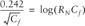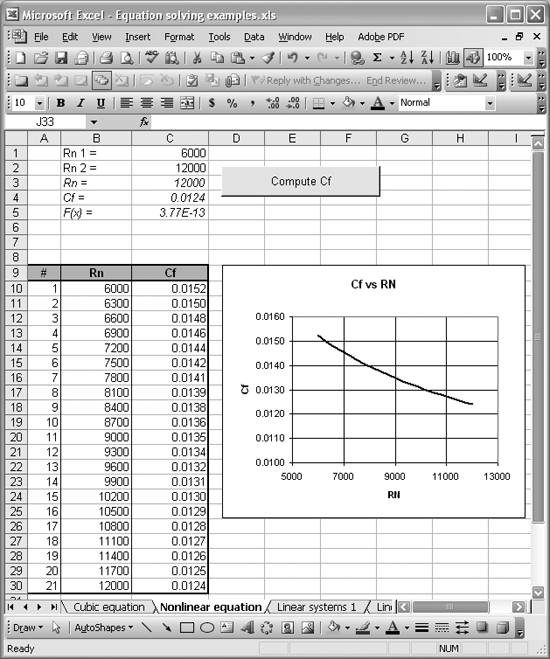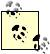# Automating Tedious Problems with VBA

Problem

You now know how to use Solver and Goal Seek to solve nonlinear equations, but the trouble is you need to solve such an equation over a range of values, making manual, repeated application of Solver and Goal Seek tedious.

Solution

Automate this process using VBA, where you can programmatically call Solver or Goal Seek.

Discussion

Solver and Goal Seek are very handy tools for solving a wide variety of problems in Excel. What's even cooler is that you can use these tools directly in VBA code, without ever having to manually open their respective windows, click cells, and so on. This means you can automate many tasks that require Solver or Goal Seek, saving yourself a lot of time.

Let's reconsider the example equation from the Recipe 9.2. The equation is repeated here for convenience:What if you wanted to plot this equation? If you wanted to plot a curve of Cf versus RN, you'd have to use Solver or Goal Seek a number of times for each point along the curve you intend to plot. Maybe you don't want to plot this curve, but instead want to generate a lookup table with Cf over a range of RN values. In either case, what a pain it would be to have to manually apply Solver or Goal Seek repeatedly for every value of RN. Imagine an even more complicated case where there's another parameter in the equation and you want to vary it systematically as well. Now you're really taking about tedium!

Thankfully, the developers of Solver and Goal Seek have exposed their functionality to us in VBA so we can automate their use.

Automating Goal Seek

To show you how to automate Goal Seek, I'll start with the spreadsheet shown in Figure 9-7 and make some modifications to it. What I want to do is add a VBA subroutine that makes calls to Goal Seek to compute values for Cf for a range of RN values. In order to call the VBA subroutine, I want to add a button control to the spreadsheet that can be clicked to start the solution process. Also, I want to set up a table that will be filled in automatically with the Cf and RN values as they are computed. Finally, I'd like to add a chart to show the resulting curve. The modified spreadsheet is shown in Figure 9-10.

Figure 9-10. Goal Seek automation exampleAs you can see, there are quite a few additions here, so I'll explain each one. The original spreadsheet elements are shown in italics in Figure 9-10; everything else is new for this example.

The first thing I added were entries in cells C1 and C2 to store starting and ending values for the range of RN values I wanted to consider. I also added text labels to the left of these cells.

Then I constructed a table in cells A9 through C30 to hold values of Cf versus RN. Initially, this table contained only the headings in row 9 and row number labels in column A. The Cf and RN cells were left blank because they will get filled in later with a custom VBA subroutine that I'll show you shortly.

With this table set up, I then created a chart to plot Cf versus RN. Since the table is initially empty, the chart didn't display any curve. However, having it set up ahead of time means that when the custom VBA subroutine is finished computing the required data, the chart will automatically be updated, showing the resulting curve. (Refer to Chapter 4 to learn how to prepare charts in Excel if you need a refresher.)

The final element to add is the button shown in Figure 9-10, labeled Compute Cf. Excel allows you to add several standard windows controls to spreadsheets, enabling you to prepare fairly rich GUI (graphical user interface) forms like the ones you see in standard Windows applications. For the purposes of this example, I'll show you how to add a simple button control and associate it with some VBA code, so that when the button is pressed it will actually do something. In this case, the VBA code will compute Cf over a range of RN and fill in the data table.

To add the button, you need to make sure the Control Toolbox toolbar is displayed in Excel. The Control Toolbox toolbar is shown in Figure 9-11.

Figure 9-11. Control Toolbox toolbarIf you don't see it, select ViewToolbars [Image_Link]/images/U2192.jpg border=0> Control Toolbox from Excels main menu bar.

To add a button, control-click the Command Button icon (see Figure 9-11) and then click anywhere in your spreadsheet where you'd like to place the button. Upon doing so, you should see a new button on your spreadsheet. Initially, it will have some resizing handles (circles) located around it so you can click and drag to resize the button. You can also click and drag the button itself to relocate it.

Initially, when you place a new control on a spreadsheet, Excel enters design mode and the Design Mode button on the Control Toolbox toolbar is depressed to indicate this. In design mode you can resize and move controls, whereas when you're not in design mode, clicking on a control will activate it. You can toggle design mode by pressing the Design Mode button on the toolbar.The controls available from the Control Toolbox are ActiveX controls. While in design mode, you can right-click on the control and select Properties from the pop-up menu to open a window displaying all of the control's properties. You can edit many characteristics of the control from the properties window. For example, the Caption property allows you to change the text shown on the control itself. In this example, I changed the caption to Compute Cf, as you can see in Figure 9-10.

With the button added, you need to associate it with some VBA code so that it will actually do something when you click it. So the next step is to add a custom VBA subroutine and then associate it with the new button.

Example 9-1 shows the custom VBA subroutine I prepared for this example. (Refer to Chapter 2 to learn how to add VBA subroutines such as this one to your spreadsheets.)

Example 9-1. ComputeCf

 ```Public Sub ComputeCf( ) Dim inc As Double With Worksheets("Nonlinear equation") inc = (.Range("Rn_2") - .Range("Rn_1")) / 20 For i = 0 To 20 .Range("Rn") = .Range("Rn_1") + (inc * i) .Range("Fx").GoalSeek goal:=0, ChangingCell:=.Range("Cf") .Cells(10 + i, 2) = .Range("Rn") .Cells(10 + i, 3) = .Range("Cf") Next i End With End Sub ```

This subroutine, called ComputeCf, exTRacts the RN range from cells C1 and C2 in the spreadsheet and then computes 20 corresponding values of Cf using Goal Seek. As each Cf value is computed, the results are placed in the table contained in cells A9 through C30 of the spreadsheet.

The first line of code within the subroutine declares a local variable, inc, that is the increment (to be computed later) between successive RN values in the range.

The next statement is a With statement. With statements are useful in object-oriented languages such as VBA where members of objects are accessed with the . syntax that you see in this example. With statements allow you to specify which object to use as the default object when accessing members. This way you can leave off the parent object name, saving yourself some typing. For example, here I'm using the statement With Worksheets("Nonlinear equation"). I'm telling VBA that I want access to the worksheet object named "Nonlinear equation." That's the name I gave to the worksheet we're using in this example. You can see this name in the worksheet tab at the bottom of Figure 9-10. Now, every time I use a statement like .Range("Rn_2"), in this case accessing the range object, VBA defaults to accessing the range object of the Nonlinear equation worksheet object. Without using the With statement, you'd have to write Worksheets("Nonlinear equation").Range("Rn_2").With statements must be bracketed with an End With statement, as shown in Example 9-1. This limits the scope of the With statement to apply to everything within the With and End With statements.

The next statement after the With statement computes inc by accessing the cells via range objects containing the starting and ending RN values, as discussed earlier. Notice that I'm using the name labels Rn_2 and Rn_1 here to access the cells. I find names far more readable than standard cell references. Take a look at Recipe 1.14 to learn how to add cell names like these.

After inc is computed, the subroutine enters a for loop iterating over the range of RN values. For each RN, Goal Seek is used to iteratively find a corresponding Cf value. Let me explain each line of the for loop:

.Range("Rn") = .Range("Rn_1") + (inc * i)

This line computes the next RN value and stores it in the cell named Rn (cell C3).

.Range("Fx").GoalSeek goal:=0, ChangingCell:=.Range("Cf")

This is the line that actually calls Goal Seek for the cell named Fx (cell C4). Fx is the target cell whose value we want to set to 0. In the VBA code shown here, the target value is specified by goal:=0. We also specify the By Changing Cell with ChangingCell:=.Range("Cf"), telling Goal Seek to vary Cf until Fx is zero.

.Cells(10 + i, 2) = .Range("Rn")

This line adds the Rn value for this iteration in our data table starting at cell B10 and incrementing by 1 row at each iteration through the loop. Notice here that I'm using R1C1-style cell references for convenience. (See Recipe 1.6 for more information on this style.)

.Cells(10 + i, 3) = .Range("Cf")

This line adds the Cf value found by Goal Seek to the data table. Here again, I'm using the R1C1 reference style.

With the ComputeCf subroutine written, you can now associate it with the button we added earlier. Enter design mode for the control as discussed earlier and then right-click on the button to reveal a pop-up menu. Select View Code from this menu. This will switch you over to the VBA editor, where you should see a new subroutine for this button. Our new subroutine is shown in Example 9-2.

Example 9-2. New button subroutine

 ```Private Sub CommandButton1_Click( ) End Sub ```

This is the click event handler for the button you added earlier. The button's default name is CommandButton1, so this subroutine is named CommandButton1_Click.You can change the name of a control by changing the Name property in the ActiveX control's property window. In design mode, right-click on the control and select Properties from the pop-up menu to display the Properties window. You can then make the appropriate changes to the control's properties.

Initially, this subroutine is empty, so you need to add a call to our custom subroutine, ComputeCf, as shown in Example 9-3.

Example 9-3. Add call to ComputeCf

 ```Private Sub CommandButton1_Click( ) ComputeCf End Sub ```

When you click the button in Excel now (with design mode off), it will execute the custom subroutine, computing Cf values over the desired RN range. You'll also see the results displayed in the table and on the chart. Should you decide you want to compute new Cf values over a different range of RN values, you need only enter the new range in cells C1 and C2 and press the button again.Excel has another set of form controls available from the Forms toolbar (select View [Image_Link]/images/U2192.jpg border=0> Toolbars [Image_Link]/images/U2192.jpg border=0> Forms to show it). These controls look like the ActiveX controls we used in this example, but give you more control. The Form controls work a little differently in terms of how you edit their properties and assign functionality to them. In general, the ActiveX controls are newer and more powerful, while the Forms controls are older and maintained for backward compatibility.

Automating Solver

You can automate Solver in a manner similar to the way in which we automated Goal Seek earlier. In fact, we can modify the earlier example slightly to use Solver instead of Goal Seek. All of the spreadsheet elements can remain the same as before and we need only make some VBA code modifications in the ComputeCf subroutine. The new ComputeCf subroutine is shown in Example 9-4.

Example 9-4. ComputeCf using Solver

 ```Public Sub ComputeCf( ) Dim inc As Double With Worksheets("Nonlinear equation") inc = (.Range("Rn_2") - .Range("Rn_1")) / 20 For i = 0 To 20 .Range("Rn") = .Range("Rn_1") + (inc * i) ' New code starts here: .Range("Cf") = 0.001 SolverOK SetCell:=Range("Fx"), MaxMinVal:=3, ValueOf:=0, _ ByChange:=Range("Cf") SolverSolve UserFinish:=True SolverFinish KeepFinal:=1 .Cells(10 + i, 2) = .Range("Rn") .Cells(10 + i, 3) = .Range("Cf") Next i End With End Sub ```

The new code consists of the four lines of code following the ' New code starts here: comment. The new lines are:

.Range("Cf") = 0.001

This line sets the initial value for Cf to 0.001. This serves as our initial guess prior to calling Solver.

SolverOK SetCell:=Range("Fx"), MaxMinVal:=3, ValueOf:=0, ByChange:=Range("Cf")

This line calls the Solver subroutine SolverOK to initialize the Solver model. The target cell is set to Fx by SetCell:=Range("Fx"). We want to set it to a specific value, so we set MaxMinVal:=3. If we wanted to maximize the target, we'd set MaxMinVal to 1; if we wanted to minimize it, we'd set MaxMinVal to 2. The target value is set via ValueOf:=0, which tells Solver to set the target value to 0. ByChange:=Range("Cf") tells Solver to vary the value in the cell named Cf to find a solution.

SolverSolve UserFinish:=True

Calling SolverSolve actually starts Solver. We specify UserFinish:=True to let Solver know that we do not want it to display the Results dialog box every time it finds a solution. If we set this to false, Solver will display the Results dialog, which can be useful for debugging.

SolverFinish KeepFinal:=1

This line tells Solver that we want to keep the results it finds.

Upon making these code changes and running the code by pressing the button on the example spreadsheet, you should see results that are very similar to those obtained using Goal Seek.If after adding the new code you attempt to run the subroutine and get a "Sub or Function not Defined" error, you need to make sure Solver is being referenced in your VBA editor. To do so, in the VBA editor select Tools [Image_Link]/images/U2192.jpg border=0> References...from the main menu bar. In the References window that appears, make sure SOLVER is checked and press OK.

You can control many more aspects of Solver using VBA code than those I showed here. For more information, see the Solver help in Excel and check out Microsoft's support web site, http://support.microsoft.com, where you can search for technical articles on Solver (among many other Microsoft product-related topics). The article "How to create Visual Basic macros by using Excel Solver in Excel 97" (Article ID 843304) should get you well on your way to becoming a Solver power user.Excel Scientific and Engineering Cookbook (Cookbooks (OReilly))
ISBN: 0596008791
EAN: 2147483647
Year: N/A
Pages: 206
Authors: David M Bourg